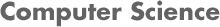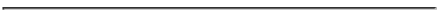### CS534 Artificial Intelligence Homework 5 - Cisco Spring 2013

#### PROF. CAROLINA RUIZ

Due Date: May 21, 2013 at the beginning of class• Instructions:
• Read Chapters/Sections 10, 11.1, 13, 14.1,14.2,14.4.1, 15.1 of the textbook.
• See multiple examples of planning problems in the solutions to homework and exam problems on the webpages of my offerings of CS4341 (ugrad AI).
• This is an individual homework. Please state in your homework submission any sources you used in constructing your solutions, as well as whom you discussed these homework problems with.

• Problems: Turn in written solutions to each of the problems below at the beginning of class when the homework is due.

1. Problem 1:

Solutions: See Andrew Sawchuk's final plan obtained in his solutions to this problem.

Consider the following planning problem discussed in the textbook's slides and in class:

• Initial situation: At(Home) ∧ Sells(Supermarket,Milk) ∧ Sells(Supermarker,Bananas) ∧ Sells(HardwareStore,Drill)

• Final situation: Have(Milk) ∧ Have(Bananas) ∧ Have(Drill) ∧ At(Home)

• Actions:

• Action: Go(p,q)
Precondition: At(p)
Effect: At(q) ∧ ¬At(p)

Precondition: At(p) ∧ Sells(p,x)
Effect: Have(x)

Use the backward planning algorithm discussed in class to construct a plan to solve this problem. At each point in the construction of the plan, explicitly state:

1. (2 points) what open condition you select;
2. (10 points) how you fulfill that condition (either by the initial situation, an action already present in the plan, or a new action);
3. (2 points) what variable instantiations are needed;
4. (10 points) whether or not order constraints (promotion or demotion) between a newly added action and actions currently in the plan are needed;
5. (6 points) when backtracking is needed to avoid failure.

2. Problem 2: (20 points) Read Section 11.1 (Time, Schedules, and Resources) in the Russell's and Norvig's textbook. Write an explicatory summary (couple of paragraphs) highlighting the main points covered in that section.

3. Problem 3:

Solutions: See Prof. Ruiz's solutions to this problem.

Consider the Bayesian net in Figure 14.21 (p. 560) of the Russell's and Norvig's textbook. Assume the prior and conditional probabilities given below:

• P(B) = 0.95
• P(R|B) = 0.8; P(R|¬B) = 0
• P(I|B) = 0.98; P(I|¬B) = 0
• P(G) = 0.65
• P(S|I∧G)=0.9; P(S|I∧¬G)=0; P(S|¬I∧G)=0; P(S|¬I∧¬G)=0
• P(M|S) = 0.7; P(M|¬S) = 0